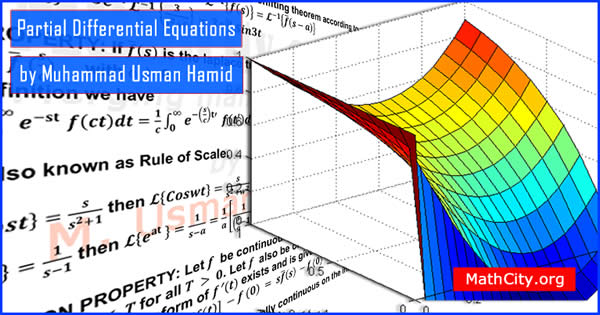# Partial Differential Equations by M Usman Hamid

The course provides a foundation to solve PDE’s with special emphasis on wave, heat and Laplace equations, formulation and some theory of these equations are also intended. We are really very thankful to Prof. Muhammad Usman Hamid for providing these notes and appreciates his effort to publish these notes on MathCity.orgName Partial Differential Equations (PDE’s) Muhammad Usman Hamid 113 pages PDF (see Software section for PDF Reader) 2.22 MB
• INTRODUCTION
• Basic Concepts and Definitions, Superposition Principle, Exercises
• FIRST-ORDER QUASI-LINEAR EQUATIONS AND METHOD OF CHARACTERISTICS
• Classification of first-order equations, Construction of a First-Order Equation, Method of Characteristics and General Solutions, Canonical Forms of First-Order Linear Equations, Method of Separation of Variables, Exercises
• MATHEMATICAL MODELS
• Heat Equations and consequences, Wave Equations and consequences.
• CLASSIFICATION OF SECOND-ORDER LINEAR EQUATIONS
• Second-Order Equations in Two Independent Variables, Canonical Forms, Equations with Constant Coefficients, General Solutions, Summary and Further Simplification, Exercises
• FOURIER SERIES, FOURIER TRANSFORMATION AND INTEGRALS WITH APPLICATIONS
• Introduction, Fourier Transform, Properties of Fourier Transform , Convolution theorem, Fourier Sine and Fourier Cosine, Exercises, Fourier Series and its complex form
• LAPLACE TRANSFORMS
• Properties of Laplace Transforms, Convolution Theorem of the Laplace Transform, Laplace Transforms of the Heaviside and Dirac Delta Functions, Hankel Transforms, Properties of Hankel Transforms and Applications, Few results about finite fourier transforms, Exercises

Please click on View Online to see inside the PDF.

• msc/notes/partial-differential-equations-m-usman-hamid